Point Cloud Library (PCL)  1.13.1-dev
pcl::NormalDistributionsTransform< PointSource, PointTarget, Scalar > Class Template Reference

A 3D Normal Distribution Transform registration implementation for point cloud data. More...

#include <pcl/registration/ndt.h>Inheritance diagram for pcl::NormalDistributionsTransform< PointSource, PointTarget, Scalar >:Collaboration diagram for pcl::NormalDistributionsTransform< PointSource, PointTarget, Scalar >:

## Public Types

using Ptr = shared_ptr< NormalDistributionsTransform< PointSource, PointTarget, Scalar > >

using ConstPtr = shared_ptr< const NormalDistributionsTransform< PointSource, PointTarget, Scalar > >

using Vector3 = typename Eigen::Matrix< Scalar, 3, 1 >

using Matrix4 = typename Registration< PointSource, PointTarget, Scalar >::Matrix4

using Affine3 = typename Eigen::Transform< Scalar, 3, Eigen::Affine >Public Types inherited from pcl::Registration< PointSource, PointTarget, float >
using Matrix4 = Eigen::Matrix< float, 4, 4 >

using Ptr = shared_ptr< Registration< PointSource, PointTarget, float > >

using ConstPtr = shared_ptr< const Registration< PointSource, PointTarget, float > >

using CorrespondenceRejectorPtr = pcl::registration::CorrespondenceRejector::Ptr

using KdTree = pcl::search::KdTree< PointTarget >

using KdTreePtr = typename KdTree::Ptr

using KdTreeReciprocal = pcl::search::KdTree< PointSource >

using KdTreeReciprocalPtr = typename KdTreeReciprocal::Ptr

using PointCloudSource = pcl::PointCloud< PointSource >

using PointCloudSourcePtr = typename PointCloudSource::Ptr

using PointCloudSourceConstPtr = typename PointCloudSource::ConstPtr

using PointCloudTarget = pcl::PointCloud< PointTarget >

using PointCloudTargetPtr = typename PointCloudTarget::Ptr

using PointCloudTargetConstPtr = typename PointCloudTarget::ConstPtr

using PointRepresentationConstPtr = typename KdTree::PointRepresentationConstPtr

using TransformationEstimation = typename pcl::registration::TransformationEstimation< PointSource, PointTarget, float >

using TransformationEstimationPtr = typename TransformationEstimation::Ptr

using TransformationEstimationConstPtr = typename TransformationEstimation::ConstPtr

using CorrespondenceEstimation = pcl::registration::CorrespondenceEstimationBase< PointSource, PointTarget, float >

using CorrespondenceEstimationPtr = typename CorrespondenceEstimation::Ptr

using CorrespondenceEstimationConstPtr = typename CorrespondenceEstimation::ConstPtr

using UpdateVisualizerCallbackSignature = void(const pcl::PointCloud< PointSource > &, const pcl::Indices &, const pcl::PointCloud< PointTarget > &, const pcl::Indices &)
The callback signature to the function updating intermediate source point cloud position during it's registration to the target point cloud. More...Public Types inherited from pcl::PCLBase< PointSource >
using PointCloud = pcl::PointCloud< PointSource >

using PointCloudPtr = typename PointCloud::Ptr

using PointCloudConstPtr = typename PointCloud::ConstPtr

using PointIndicesPtr = PointIndices::Ptr

using PointIndicesConstPtr = PointIndices::ConstPtr

## Public Member Functions

NormalDistributionsTransform ()
Constructor. More...

~NormalDistributionsTransform () override=default
Empty destructor. More...

void setInputTarget (const PointCloudTargetConstPtr &cloud) override
Provide a pointer to the input target (e.g., the point cloud that we want to align the input source to). More...

void setResolution (float resolution)
Set/change the voxel grid resolution. More...

void setMinPointPerVoxel (unsigned int min_points_per_voxel)
Set the minimum number of points required for a cell to be used (must be 3 or greater for covariance calculation). More...

float getResolution () const
Get voxel grid resolution. More...

double getStepSize () const
Get the newton line search maximum step length. More...

void setStepSize (double step_size)
Set/change the newton line search maximum step length. More...

double getOulierRatio () const
Get the point cloud outlier ratio. More...

void setOulierRatio (double outlier_ratio)
Set/change the point cloud outlier ratio. More...

double getTransformationLikelihood () const
Get the registration alignment likelihood. More...

double getTransformationProbability () const
Get the registration alignment probability. More...

int getFinalNumIteration () const
Get the number of iterations required to calculate alignment. More...Public Member Functions inherited from pcl::Registration< PointSource, PointTarget, float >
Registration ()
Empty constructor. More...

~Registration () override=default
destructor. More...

void setTransformationEstimation (const TransformationEstimationPtr &te)
Provide a pointer to the transformation estimation object. More...

void setCorrespondenceEstimation (const CorrespondenceEstimationPtr &ce)
Provide a pointer to the correspondence estimation object. More...

virtual void setInputSource (const PointCloudSourceConstPtr &cloud)
Provide a pointer to the input source (e.g., the point cloud that we want to align to the target) More...

PointCloudSourceConstPtr const getInputSource ()
Get a pointer to the input point cloud dataset target. More...

PointCloudTargetConstPtr const getInputTarget ()
Get a pointer to the input point cloud dataset target. More...

void setSearchMethodTarget (const KdTreePtr &tree, bool force_no_recompute=false)
Provide a pointer to the search object used to find correspondences in the target cloud. More...

KdTreePtr getSearchMethodTarget () const
Get a pointer to the search method used to find correspondences in the target cloud. More...

void setSearchMethodSource (const KdTreeReciprocalPtr &tree, bool force_no_recompute=false)
Provide a pointer to the search object used to find correspondences in the source cloud (usually used by reciprocal correspondence finding). More...

KdTreeReciprocalPtr getSearchMethodSource () const
Get a pointer to the search method used to find correspondences in the source cloud. More...

Matrix4 getFinalTransformation ()
Get the final transformation matrix estimated by the registration method. More...

Matrix4 getLastIncrementalTransformation ()
Get the last incremental transformation matrix estimated by the registration method. More...

void setMaximumIterations (int nr_iterations)
Set the maximum number of iterations the internal optimization should run for. More...

int getMaximumIterations ()
Get the maximum number of iterations the internal optimization should run for, as set by the user. More...

void setRANSACIterations (int ransac_iterations)
Set the number of iterations RANSAC should run for. More...

double getRANSACIterations ()
Get the number of iterations RANSAC should run for, as set by the user. More...

void setRANSACOutlierRejectionThreshold (double inlier_threshold)
Set the inlier distance threshold for the internal RANSAC outlier rejection loop. More...

double getRANSACOutlierRejectionThreshold ()
Get the inlier distance threshold for the internal outlier rejection loop as set by the user. More...

void setMaxCorrespondenceDistance (double distance_threshold)
Set the maximum distance threshold between two correspondent points in source <-> target. More...

double getMaxCorrespondenceDistance ()
Get the maximum distance threshold between two correspondent points in source <-> target. More...

void setTransformationEpsilon (double epsilon)
Set the transformation epsilon (maximum allowable translation squared difference between two consecutive transformations) in order for an optimization to be considered as having converged to the final solution. More...

double getTransformationEpsilon ()
Get the transformation epsilon (maximum allowable translation squared difference between two consecutive transformations) as set by the user. More...

void setTransformationRotationEpsilon (double epsilon)
Set the transformation rotation epsilon (maximum allowable rotation difference between two consecutive transformations) in order for an optimization to be considered as having converged to the final solution. More...

double getTransformationRotationEpsilon ()
Get the transformation rotation epsilon (maximum allowable difference between two consecutive transformations) as set by the user (epsilon is the cos(angle) in a axis-angle representation). More...

void setEuclideanFitnessEpsilon (double epsilon)
Set the maximum allowed Euclidean error between two consecutive steps in the ICP loop, before the algorithm is considered to have converged. More...

double getEuclideanFitnessEpsilon ()
Get the maximum allowed distance error before the algorithm will be considered to have converged, as set by the user. More...

void setPointRepresentation (const PointRepresentationConstPtr &point_representation)
Provide a boost shared pointer to the PointRepresentation to be used when comparing points. More...

bool registerVisualizationCallback (std::function< UpdateVisualizerCallbackSignature > &visualizerCallback)
Register the user callback function which will be called from registration thread in order to update point cloud obtained after each iteration. More...

double getFitnessScore (double max_range=std::numeric_limits< double >::max())
Obtain the Euclidean fitness score (e.g., mean of squared distances from the source to the target) More...

double getFitnessScore (const std::vector< float > &distances_a, const std::vector< float > &distances_b)
Obtain the Euclidean fitness score (e.g., mean of squared distances from the source to the target) from two sets of correspondence distances (distances between source and target points) More...

bool hasConverged () const
Return the state of convergence after the last align run. More...

void align (PointCloudSource &output)
Call the registration algorithm which estimates the transformation and returns the transformed source (input) as output. More...

void align (PointCloudSource &output, const Matrix4 &guess)
Call the registration algorithm which estimates the transformation and returns the transformed source (input) as output. More...

const std::string & getClassName () const
Abstract class get name method. More...

bool initCompute ()
Internal computation initialization. More...

bool initComputeReciprocal ()
Internal computation when reciprocal lookup is needed. More...

Add a new correspondence rejector to the list. More...

std::vector< CorrespondenceRejectorPtrgetCorrespondenceRejectors ()
Get the list of correspondence rejectors. More...

bool removeCorrespondenceRejector (unsigned int i)
Remove the i-th correspondence rejector in the list. More...

void clearCorrespondenceRejectors ()
Clear the list of correspondence rejectors. More...Public Member Functions inherited from pcl::PCLBase< PointSource >
PCLBase ()
Empty constructor. More...

PCLBase (const PCLBase &base)
Copy constructor. More...

virtual ~PCLBase ()=default
Destructor. More...

virtual void setInputCloud (const PointCloudConstPtr &cloud)
Provide a pointer to the input dataset. More...

PointCloudConstPtr const getInputCloud () const
Get a pointer to the input point cloud dataset. More...

virtual void setIndices (const IndicesPtr &indices)
Provide a pointer to the vector of indices that represents the input data. More...

virtual void setIndices (const IndicesConstPtr &indices)
Provide a pointer to the vector of indices that represents the input data. More...

virtual void setIndices (const PointIndicesConstPtr &indices)
Provide a pointer to the vector of indices that represents the input data. More...

virtual void setIndices (std::size_t row_start, std::size_t col_start, std::size_t nb_rows, std::size_t nb_cols)
Set the indices for the points laying within an interest region of the point cloud. More...

IndicesPtr getIndices ()
Get a pointer to the vector of indices used. More...

IndicesConstPtr const getIndices () const
Get a pointer to the vector of indices used. More...

const PointSource & operator[] (std::size_t pos) const
Override PointCloud operator[] to shorten code. More...

## Static Public Member Functions

static void convertTransform (const Eigen::Matrix< double, 6, 1 > &x, Affine3 &trans)
Convert 6 element transformation vector to affine transformation. More...

static void convertTransform (const Eigen::Matrix< double, 6, 1 > &x, Matrix4 &trans)
Convert 6 element transformation vector to transformation matrix. More...

## Protected Types

using PointCloudSource = typename Registration< PointSource, PointTarget, Scalar >::PointCloudSource

using PointCloudSourcePtr = typename PointCloudSource::Ptr

using PointCloudSourceConstPtr = typename PointCloudSource::ConstPtr

using PointCloudTarget = typename Registration< PointSource, PointTarget, Scalar >::PointCloudTarget

using PointCloudTargetPtr = typename PointCloudTarget::Ptr

using PointCloudTargetConstPtr = typename PointCloudTarget::ConstPtr

using PointIndicesPtr = PointIndices::Ptr

using PointIndicesConstPtr = PointIndices::ConstPtr

using TargetGrid = VoxelGridCovariance< PointTarget >
Typename of searchable voxel grid containing mean and covariance. More...

using TargetGridPtr = TargetGrid *
Typename of pointer to searchable voxel grid. More...

using TargetGridConstPtr = const TargetGrid *
Typename of const pointer to searchable voxel grid. More...

using TargetGridLeafConstPtr = typename TargetGrid::LeafConstPtr
Typename of const pointer to searchable voxel grid leaf. More...

## Protected Member Functions

virtual void computeTransformation (PointCloudSource &output)
Estimate the transformation and returns the transformed source (input) as output. More...

void computeTransformation (PointCloudSource &output, const Matrix4 &guess) override
Estimate the transformation and returns the transformed source (input) as output. More...

void init ()
Initiate covariance voxel structure. More...

double computeDerivatives (Eigen::Matrix< double, 6, 1 > &score_gradient, Eigen::Matrix< double, 6, 6 > &hessian, const PointCloudSource &trans_cloud, const Eigen::Matrix< double, 6, 1 > &transform, bool compute_hessian=true)
Compute derivatives of likelihood function w.r.t. More...

double updateDerivatives (Eigen::Matrix< double, 6, 1 > &score_gradient, Eigen::Matrix< double, 6, 6 > &hessian, const Eigen::Vector3d &x_trans, const Eigen::Matrix3d &c_inv, bool compute_hessian=true) const
Compute individual point contributions to derivatives of likelihood function w.r.t. More...

void computeAngleDerivatives (const Eigen::Matrix< double, 6, 1 > &transform, bool compute_hessian=true)
Precompute angular components of derivatives. More...

void computePointDerivatives (const Eigen::Vector3d &x, bool compute_hessian=true)
Compute point derivatives. More...

void computeHessian (Eigen::Matrix< double, 6, 6 > &hessian, const PointCloudSource &trans_cloud)
Compute hessian of likelihood function w.r.t. More...

void computeHessian (Eigen::Matrix< double, 6, 6 > &hessian, const PointCloudSource &trans_cloud, const Eigen::Matrix< double, 6, 1 > &transform)
Compute hessian of likelihood function w.r.t. More...

void updateHessian (Eigen::Matrix< double, 6, 6 > &hessian, const Eigen::Vector3d &x_trans, const Eigen::Matrix3d &c_inv) const
Compute individual point contributions to hessian of likelihood function w.r.t. More...

double computeStepLengthMT (const Eigen::Matrix< double, 6, 1 > &transform, Eigen::Matrix< double, 6, 1 > &step_dir, double step_init, double step_max, double step_min, double &score, Eigen::Matrix< double, 6, 1 > &score_gradient, Eigen::Matrix< double, 6, 6 > &hessian, PointCloudSource &trans_cloud)
Compute line search step length and update transform and likelihood derivatives using More-Thuente method. More...

bool updateIntervalMT (double &a_l, double &f_l, double &g_l, double &a_u, double &f_u, double &g_u, double a_t, double f_t, double g_t) const
Update interval of possible step lengths for More-Thuente method,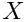in More-Thuente (1994) More...

double trialValueSelectionMT (double a_l, double f_l, double g_l, double a_u, double f_u, double g_u, double a_t, double f_t, double g_t) const
Select new trial value for More-Thuente method. More...

double auxilaryFunction_PsiMT (double a, double f_a, double f_0, double g_0, double mu=1.e-4) const
Auxiliary function used to determine endpoints of More-Thuente interval. More...

double auxilaryFunction_dPsiMT (double g_a, double g_0, double mu=1.e-4) const
Auxiliary function derivative used to determine endpoints of More-Thuente interval. More...Protected Member Functions inherited from pcl::Registration< PointSource, PointTarget, float >
bool searchForNeighbors (const PointCloudSource &cloud, int index, pcl::Indices &indices, std::vector< float > &distances)
Search for the closest nearest neighbor of a given point. More...

virtual void computeTransformation (PointCloudSource &output, const Matrix4 &guess)=0
Abstract transformation computation method with initial guess. More...Protected Member Functions inherited from pcl::PCLBase< PointSource >
bool initCompute ()
This method should get called before starting the actual computation. More...

bool deinitCompute ()
This method should get called after finishing the actual computation. More...

## Protected Attributes

TargetGrid target_cells_
The voxel grid generated from target cloud containing point means and covariances. More...

float resolution_
The side length of voxels. More...

double step_size_
The maximum step length. More...

double outlier_ratio_
The ratio of outliers of points w.r.t. More...

double gauss_d1_
The normalization constants used fit the point distribution to a normal distribution, Equation 6.8 [Magnusson 2009]. More...

double gauss_d2_

union {
double   trans_probability_

double   trans_likelihood_

};
The likelihood score of the transform applied to the input cloud, Equation 6.9 and 6.10 [Magnusson 2009]. More...

Eigen::Matrix< double, 8, 4 > angular_jacobian_

Eigen::Matrix< double, 15, 4 > angular_hessian_
Precomputed Angular Hessian. More...

Eigen::Matrix< double, 3, 6 > point_jacobian_
The first order derivative of the transformation of a point w.r.t. More...

Eigen::Matrix< double, 18, 6 > point_hessian_
The second order derivative of the transformation of a point w.r.t. More...Protected Attributes inherited from pcl::Registration< PointSource, PointTarget, float >
std::string reg_name_
The registration method name. More...

KdTreePtr tree_
A pointer to the spatial search object. More...

KdTreeReciprocalPtr tree_reciprocal_
A pointer to the spatial search object of the source. More...

int nr_iterations_
The number of iterations the internal optimization ran for (used internally). More...

int max_iterations_
The maximum number of iterations the internal optimization should run for. More...

int ransac_iterations_
The number of iterations RANSAC should run for. More...

PointCloudTargetConstPtr target_
The input point cloud dataset target. More...

Matrix4 final_transformation_
The final transformation matrix estimated by the registration method after N iterations. More...

Matrix4 transformation_
The transformation matrix estimated by the registration method. More...

Matrix4 previous_transformation_
The previous transformation matrix estimated by the registration method (used internally). More...

double transformation_epsilon_
The maximum difference between two consecutive transformations in order to consider convergence (user defined). More...

double transformation_rotation_epsilon_
The maximum rotation difference between two consecutive transformations in order to consider convergence (user defined). More...

double euclidean_fitness_epsilon_
The maximum allowed Euclidean error between two consecutive steps in the ICP loop, before the algorithm is considered to have converged. More...

double corr_dist_threshold_
The maximum distance threshold between two correspondent points in source <-> target. More...

double inlier_threshold_
The inlier distance threshold for the internal RANSAC outlier rejection loop. More...

bool converged_
Holds internal convergence state, given user parameters. More...

unsigned int min_number_correspondences_
The minimum number of correspondences that the algorithm needs before attempting to estimate the transformation. More...

CorrespondencesPtr correspondences_
The set of correspondences determined at this ICP step. More...

TransformationEstimationPtr transformation_estimation_
A TransformationEstimation object, used to calculate the 4x4 rigid transformation. More...

CorrespondenceEstimationPtr correspondence_estimation_
A CorrespondenceEstimation object, used to estimate correspondences between the source and the target cloud. More...

std::vector< CorrespondenceRejectorPtrcorrespondence_rejectors_
The list of correspondence rejectors to use. More...

bool target_cloud_updated_
Variable that stores whether we have a new target cloud, meaning we need to pre-process it again. More...

bool source_cloud_updated_
Variable that stores whether we have a new source cloud, meaning we need to pre-process it again. More...

bool force_no_recompute_
A flag which, if set, means the tree operating on the target cloud will never be recomputed. More...

bool force_no_recompute_reciprocal_
A flag which, if set, means the tree operating on the source cloud will never be recomputed. More...

std::function< UpdateVisualizerCallbackSignatureupdate_visualizer_
Callback function to update intermediate source point cloud position during it's registration to the target point cloud. More...Protected Attributes inherited from pcl::PCLBase< PointSource >
PointCloudConstPtr input_
The input point cloud dataset. More...

IndicesPtr indices_
A pointer to the vector of point indices to use. More...

bool use_indices_
Set to true if point indices are used. More...

bool fake_indices_
If no set of indices are given, we construct a set of fake indices that mimic the input PointCloud. More...

## Detailed Description

### template<typename PointSource, typename PointTarget, typename Scalar = float> class pcl::NormalDistributionsTransform< PointSource, PointTarget, Scalar >

A 3D Normal Distribution Transform registration implementation for point cloud data.

Note
For more information please see Magnusson, M. (2009). The Three-Dimensional Normal-Distributions Transform — an Efﬁcient Representation for Registration, Surface Analysis, and Loop Detection. PhD thesis, Orebro University. Orebro Studies in Technology 36., More, J., and Thuente, D. (1994). Line Search Algorithm with Guaranteed Sufficient Decrease In ACM Transactions on Mathematical Software. and Sun, W. and Yuan, Y, (2006) Optimization Theory and Methods: Nonlinear Programming. 89-100
Math refactored by Todor Stoyanov.

Definition at line 65 of file ndt.h.

## ◆ Affine3

template<typename PointSource , typename PointTarget , typename Scalar = float>
 using pcl::NormalDistributionsTransform< PointSource, PointTarget, Scalar >::Affine3 = typename Eigen::Transform

Definition at line 98 of file ndt.h.

## ◆ ConstPtr

template<typename PointSource , typename PointTarget , typename Scalar = float>
 using pcl::NormalDistributionsTransform< PointSource, PointTarget, Scalar >::ConstPtr = shared_ptr >

Definition at line 94 of file ndt.h.

## ◆ Matrix4

template<typename PointSource , typename PointTarget , typename Scalar = float>
 using pcl::NormalDistributionsTransform< PointSource, PointTarget, Scalar >::Matrix4 = typename Registration::Matrix4

Definition at line 97 of file ndt.h.

## ◆ PointCloudSource

template<typename PointSource , typename PointTarget , typename Scalar = float>
 using pcl::NormalDistributionsTransform< PointSource, PointTarget, Scalar >::PointCloudSource = typename Registration::PointCloudSource
protected

Definition at line 68 of file ndt.h.

## ◆ PointCloudSourceConstPtr

template<typename PointSource , typename PointTarget , typename Scalar = float>
 using pcl::NormalDistributionsTransform< PointSource, PointTarget, Scalar >::PointCloudSourceConstPtr = typename PointCloudSource::ConstPtr
protected

Definition at line 71 of file ndt.h.

## ◆ PointCloudSourcePtr

template<typename PointSource , typename PointTarget , typename Scalar = float>
 using pcl::NormalDistributionsTransform< PointSource, PointTarget, Scalar >::PointCloudSourcePtr = typename PointCloudSource::Ptr
protected

Definition at line 70 of file ndt.h.

## ◆ PointCloudTarget

template<typename PointSource , typename PointTarget , typename Scalar = float>
 using pcl::NormalDistributionsTransform< PointSource, PointTarget, Scalar >::PointCloudTarget = typename Registration::PointCloudTarget
protected

Definition at line 73 of file ndt.h.

## ◆ PointCloudTargetConstPtr

template<typename PointSource , typename PointTarget , typename Scalar = float>
 using pcl::NormalDistributionsTransform< PointSource, PointTarget, Scalar >::PointCloudTargetConstPtr = typename PointCloudTarget::ConstPtr
protected

Definition at line 76 of file ndt.h.

## ◆ PointCloudTargetPtr

template<typename PointSource , typename PointTarget , typename Scalar = float>
 using pcl::NormalDistributionsTransform< PointSource, PointTarget, Scalar >::PointCloudTargetPtr = typename PointCloudTarget::Ptr
protected

Definition at line 75 of file ndt.h.

## ◆ PointIndicesConstPtr

template<typename PointSource , typename PointTarget , typename Scalar = float>
 using pcl::NormalDistributionsTransform< PointSource, PointTarget, Scalar >::PointIndicesConstPtr = PointIndices::ConstPtr
protected

Definition at line 79 of file ndt.h.

## ◆ PointIndicesPtr

template<typename PointSource , typename PointTarget , typename Scalar = float>
 using pcl::NormalDistributionsTransform< PointSource, PointTarget, Scalar >::PointIndicesPtr = PointIndices::Ptr
protected

Definition at line 78 of file ndt.h.

## ◆ Ptr

template<typename PointSource , typename PointTarget , typename Scalar = float>
 using pcl::NormalDistributionsTransform< PointSource, PointTarget, Scalar >::Ptr = shared_ptr >

Definition at line 92 of file ndt.h.

## ◆ TargetGrid

template<typename PointSource , typename PointTarget , typename Scalar = float>
 using pcl::NormalDistributionsTransform< PointSource, PointTarget, Scalar >::TargetGrid = VoxelGridCovariance
protected

Typename of searchable voxel grid containing mean and covariance.

Definition at line 83 of file ndt.h.

## ◆ TargetGridConstPtr

template<typename PointSource , typename PointTarget , typename Scalar = float>
 using pcl::NormalDistributionsTransform< PointSource, PointTarget, Scalar >::TargetGridConstPtr = const TargetGrid*
protected

Typename of const pointer to searchable voxel grid.

Definition at line 87 of file ndt.h.

## ◆ TargetGridLeafConstPtr

template<typename PointSource , typename PointTarget , typename Scalar = float>
 using pcl::NormalDistributionsTransform< PointSource, PointTarget, Scalar >::TargetGridLeafConstPtr = typename TargetGrid::LeafConstPtr
protected

Typename of const pointer to searchable voxel grid leaf.

Definition at line 89 of file ndt.h.

## ◆ TargetGridPtr

template<typename PointSource , typename PointTarget , typename Scalar = float>
 using pcl::NormalDistributionsTransform< PointSource, PointTarget, Scalar >::TargetGridPtr = TargetGrid*
protected

Typename of pointer to searchable voxel grid.

Definition at line 85 of file ndt.h.

## ◆ Vector3

template<typename PointSource , typename PointTarget , typename Scalar = float>
 using pcl::NormalDistributionsTransform< PointSource, PointTarget, Scalar >::Vector3 = typename Eigen::Matrix

Definition at line 96 of file ndt.h.

## ◆ NormalDistributionsTransform()

template<typename PointSource , typename PointTarget , typename Scalar >
 pcl::NormalDistributionsTransform< PointSource, PointTarget, Scalar >::NormalDistributionsTransform

## ◆ ~NormalDistributionsTransform()

template<typename PointSource , typename PointTarget , typename Scalar = float>
 pcl::NormalDistributionsTransform< PointSource, PointTarget, Scalar >::~NormalDistributionsTransform ( )
overridedefault

Empty destructor.

## ◆ auxilaryFunction_dPsiMT()

template<typename PointSource , typename PointTarget , typename Scalar = float>
 double pcl::NormalDistributionsTransform< PointSource, PointTarget, Scalar >::auxilaryFunction_dPsiMT ( double g_a, double g_0, double mu = 1.e-4 ) const
inlineprotected

Auxiliary function derivative used to determine endpoints of More-Thuente interval.

Note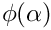, derivative of Equation 1.6 (Moore, Thuente 1994)
Parameters
 [in] g_a function gradient at step length a,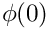in More-Thuente (1994) [in] g_0 initial function gradient,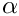in More-Thuente (1994) [in] mu the step length, constant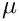in Equation 1.1 [More, Thuente 1994]
Returns
sufficient decrease derivative

Definition at line 549 of file ndt.h.

## ◆ auxilaryFunction_PsiMT()

template<typename PointSource , typename PointTarget , typename Scalar = float>
 double pcl::NormalDistributionsTransform< PointSource, PointTarget, Scalar >::auxilaryFunction_PsiMT ( double a, double f_a, double f_0, double g_0, double mu = 1.e-4 ) const
inlineprotected

Auxiliary function used to determine endpoints of More-Thuente interval.

Note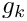in Equation 1.6 (Moore, Thuente 1994)
Parameters
 [in] a the step length,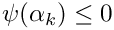in More-Thuente (1994) [in] f_a function value at step length a,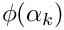in More-Thuente (1994) [in] f_0 initial function value,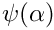in Moore-Thuente (1994) [in] g_0 initial function gradient,in More-Thuente (1994) [in] mu the step length, constantin Equation 1.1 [More, Thuente 1994]
Returns
sufficient decrease value

Definition at line 530 of file ndt.h.

## ◆ computeAngleDerivatives()

template<typename PointSource , typename PointTarget , typename Scalar >
 void pcl::NormalDistributionsTransform< PointSource, PointTarget, Scalar >::computeAngleDerivatives ( const Eigen::Matrix< double, 6, 1 > & transform, bool compute_hessian = true )
protected

Precompute angular components of derivatives.

Note
Equation 6.19 and 6.21 [Magnusson 2009].
Parameters
 [in] transform the current transform vector [in] compute_hessian flag to calculate hessian, unnecessary for step calculation.

Definition at line 242 of file ndt.hpp.

## ◆ computeDerivatives()

template<typename PointSource , typename PointTarget , typename Scalar >
 double pcl::NormalDistributionsTransform< PointSource, PointTarget, Scalar >::computeDerivatives ( Eigen::Matrix< double, 6, 1 > & score_gradient, Eigen::Matrix< double, 6, 6 > & hessian, const PointCloudSource & trans_cloud, const Eigen::Matrix< double, 6, 1 > & transform, bool compute_hessian = true )
protected

Compute derivatives of likelihood function w.r.t.

the transformation vector.

Note
Equation 6.10, 6.12 and 6.13 [Magnusson 2009].
Parameters
 [out] score_gradient the gradient vector of the likelihood function w.r.t. the transformation vector [out] hessian the hessian matrix of the likelihood function w.r.t. the transformation vector [in] trans_cloud transformed point cloud [in] transform the current transform vector [in] compute_hessian flag to calculate hessian, unnecessary for step calculation.

Definition at line 191 of file ndt.hpp.

## ◆ computeHessian() [1/2]

template<typename PointSource , typename PointTarget , typename Scalar >
 void pcl::NormalDistributionsTransform< PointSource, PointTarget, Scalar >::computeHessian ( Eigen::Matrix< double, 6, 6 > & hessian, const PointCloudSource & trans_cloud )
protected

Compute hessian of likelihood function w.r.t.

the transformation vector.

Note
Equation 6.13 [Magnusson 2009].
Parameters
 [out] hessian the hessian matrix of the likelihood function w.r.t. the transformation vector [in] trans_cloud transformed point cloud

Definition at line 421 of file ndt.hpp.

## ◆ computeHessian() [2/2]

template<typename PointSource , typename PointTarget , typename Scalar = float>
 void pcl::NormalDistributionsTransform< PointSource, PointTarget, Scalar >::computeHessian ( Eigen::Matrix< double, 6, 6 > & hessian, const PointCloudSource & trans_cloud, const Eigen::Matrix< double, 6, 1 > & transform )
inlineprotected

Compute hessian of likelihood function w.r.t.

the transformation vector.

Note
Equation 6.13 [Magnusson 2009].
Parameters
 [out] hessian the hessian matrix of the likelihood function w.r.t. the transformation vector [in] trans_cloud transformed point cloud [in] transform the current transform vector
Deprecated:
Scheduled for removal in version 1 . 15 : "Parameter transform is not required"

Definition at line 375 of file ndt.h.

## ◆ computePointDerivatives()

template<typename PointSource , typename PointTarget , typename Scalar >
 void pcl::NormalDistributionsTransform< PointSource, PointTarget, Scalar >::computePointDerivatives ( const Eigen::Vector3d & x, bool compute_hessian = true )
protected

Compute point derivatives.

Note
Equation 6.18-21 [Magnusson 2009].
Parameters
 [in] x point from the input cloud [in] compute_hessian flag to calculate hessian, unnecessary for step calculation.

Definition at line 327 of file ndt.hpp.

## ◆ computeStepLengthMT()

template<typename PointSource , typename PointTarget , typename Scalar >
 double pcl::NormalDistributionsTransform< PointSource, PointTarget, Scalar >::computeStepLengthMT ( const Eigen::Matrix< double, 6, 1 > & transform, Eigen::Matrix< double, 6, 1 > & step_dir, double step_init, double step_max, double step_min, double & score, Eigen::Matrix< double, 6, 1 > & score_gradient, Eigen::Matrix< double, 6, 6 > & hessian, PointCloudSource & trans_cloud )
protected

Compute line search step length and update transform and likelihood derivatives using More-Thuente method.

Note
Search Algorithm [More, Thuente 1994]
Parameters
 [in] transform initial transformation vector,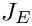in Equation 1.3 (Moore, Thuente 1994) and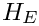in Algorithm 2 [Magnusson 2009] [in] step_dir descent direction,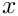in Equation 1.3 (Moore, Thuente 1994) and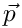normalized in Algorithm 2 [Magnusson 2009] [in] step_init initial step length estimate,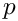in Moore-Thuente (1994) and the normal ofin Algorithm 2 [Magnusson 2009] [in] step_max maximum step length,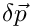in Moore-Thuente (1994) [in] step_min minimum step length,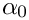in Moore-Thuente (1994) [out] score final score function value,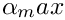in Equation 1.3 (Moore, Thuente 1994) and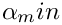in Algorithm 2 [Magnusson 2009] [in,out] score_gradient gradient of score function w.r.t. transformation vector,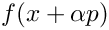in Moore-Thuente (1994) and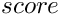in Algorithm 2 [Magnusson 2009] [out] hessian hessian of score function w.r.t. transformation vector,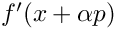in Moore-Thuente (1994) and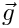in Algorithm 2 [Magnusson 2009] [in,out] trans_cloud transformed point cloud,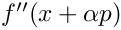transformed by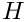in Algorithm 2 [Magnusson 2009]
Returns
final step length

Definition at line 656 of file ndt.hpp.

References pcl::transformPointCloud().

## ◆ computeTransformation() [1/2]

template<typename PointSource , typename PointTarget , typename Scalar = float>
 virtual void pcl::NormalDistributionsTransform< PointSource, PointTarget, Scalar >::computeTransformation ( PointCloudSource & output )
inlineprotectedvirtual

Estimate the transformation and returns the transformed source (input) as output.

Parameters
 [out] output the resultant input transformed point cloud dataset

Definition at line 274 of file ndt.h.

## ◆ computeTransformation() [2/2]

template<typename PointSource , typename PointTarget , typename Scalar >
 void pcl::NormalDistributionsTransform< PointSource, PointTarget, Scalar >::computeTransformation ( PointCloudSource & output, const Matrix4 & guess )
overrideprotected

Estimate the transformation and returns the transformed source (input) as output.

Parameters
 [out] output the resultant input transformed point cloud dataset [in] guess the initial gross estimation of the transformation

Definition at line 74 of file ndt.hpp.

References pcl::transformPointCloud().

## ◆ convertTransform() [1/2]

template<typename PointSource , typename PointTarget , typename Scalar = float>
 static void pcl::NormalDistributionsTransform< PointSource, PointTarget, Scalar >::convertTransform ( const Eigen::Matrix< double, 6, 1 > & x, Affine3 & trans )
inlinestatic

Convert 6 element transformation vector to affine transformation.

Parameters
 [in] x transformation vector of the form [x, y, z, roll, pitch, yaw] [out] trans affine transform corresponding to given transformation vector

Definition at line 228 of file ndt.h.

## ◆ convertTransform() [2/2]

template<typename PointSource , typename PointTarget , typename Scalar = float>
 static void pcl::NormalDistributionsTransform< PointSource, PointTarget, Scalar >::convertTransform ( const Eigen::Matrix< double, 6, 1 > & x, Matrix4 & trans )
inlinestatic

Convert 6 element transformation vector to transformation matrix.

Parameters
 [in] x transformation vector of the form [x, y, z, roll, pitch, yaw] [out] trans 4x4 transformation matrix corresponding to given transformation vector

Definition at line 242 of file ndt.h.

## ◆ getFinalNumIteration()

template<typename PointSource , typename PointTarget , typename Scalar = float>
 int pcl::NormalDistributionsTransform< PointSource, PointTarget, Scalar >::getFinalNumIteration ( ) const
inline

Get the number of iterations required to calculate alignment.

Returns
final number of iterations

Definition at line 217 of file ndt.h.

## ◆ getOulierRatio()

template<typename PointSource , typename PointTarget , typename Scalar = float>
 double pcl::NormalDistributionsTransform< PointSource, PointTarget, Scalar >::getOulierRatio ( ) const
inline

Get the point cloud outlier ratio.

Returns
outlier ratio

Definition at line 177 of file ndt.h.

## ◆ getResolution()

template<typename PointSource , typename PointTarget , typename Scalar = float>
 float pcl::NormalDistributionsTransform< PointSource, PointTarget, Scalar >::getResolution ( ) const
inline

Get voxel grid resolution.

Returns
side length of voxels

Definition at line 150 of file ndt.h.

## ◆ getStepSize()

template<typename PointSource , typename PointTarget , typename Scalar = float>
 double pcl::NormalDistributionsTransform< PointSource, PointTarget, Scalar >::getStepSize ( ) const
inline

Get the newton line search maximum step length.

Returns
maximum step length

Definition at line 159 of file ndt.h.

## ◆ getTransformationLikelihood()

template<typename PointSource , typename PointTarget , typename Scalar = float>
 double pcl::NormalDistributionsTransform< PointSource, PointTarget, Scalar >::getTransformationLikelihood ( ) const
inline

Get the registration alignment likelihood.

Returns
transformation likelihood

Definition at line 195 of file ndt.h.

## ◆ getTransformationProbability()

template<typename PointSource , typename PointTarget , typename Scalar = float>
 double pcl::NormalDistributionsTransform< PointSource, PointTarget, Scalar >::getTransformationProbability ( ) const
inline

Get the registration alignment probability.

Returns
transformation probability
Deprecated:
Scheduled for removal in version 1 . 16 : "The method getTransformationProbability has been renamed to " "getTransformationLikelihood."

Definition at line 208 of file ndt.h.

## ◆ init()

template<typename PointSource , typename PointTarget , typename Scalar = float>
 void pcl::NormalDistributionsTransform< PointSource, PointTarget, Scalar >::init ( )
inlineprotected

## ◆ setInputTarget()

template<typename PointSource , typename PointTarget , typename Scalar = float>
 void pcl::NormalDistributionsTransform< PointSource, PointTarget, Scalar >::setInputTarget ( const PointCloudTargetConstPtr & cloud )
inlineoverridevirtual

Provide a pointer to the input target (e.g., the point cloud that we want to align the input source to).

Parameters
 [in] cloud the input point cloud target

Reimplemented from pcl::Registration< PointSource, PointTarget, float >.

Definition at line 113 of file ndt.h.

## ◆ setMinPointPerVoxel()

template<typename PointSource , typename PointTarget , typename Scalar = float>
 void pcl::NormalDistributionsTransform< PointSource, PointTarget, Scalar >::setMinPointPerVoxel ( unsigned int min_points_per_voxel )
inline

Set the minimum number of points required for a cell to be used (must be 3 or greater for covariance calculation).

Calls the function of the underlying VoxelGridCovariance. This function must be called before setInputTarget and setResolution.

Parameters
 [in] min_points_per_voxel the minimum number of points required for a voxel to be used

Definition at line 141 of file ndt.h.

## ◆ setOulierRatio()

template<typename PointSource , typename PointTarget , typename Scalar = float>
 void pcl::NormalDistributionsTransform< PointSource, PointTarget, Scalar >::setOulierRatio ( double outlier_ratio )
inline

Set/change the point cloud outlier ratio.

Parameters
 [in] outlier_ratio outlier ratio

Definition at line 186 of file ndt.h.

## ◆ setResolution()

template<typename PointSource , typename PointTarget , typename Scalar = float>
 void pcl::NormalDistributionsTransform< PointSource, PointTarget, Scalar >::setResolution ( float resolution )
inline

Set/change the voxel grid resolution.

Parameters
 [in] resolution side length of voxels

Definition at line 123 of file ndt.h.

## ◆ setStepSize()

template<typename PointSource , typename PointTarget , typename Scalar = float>
 void pcl::NormalDistributionsTransform< PointSource, PointTarget, Scalar >::setStepSize ( double step_size )
inline

Set/change the newton line search maximum step length.

Parameters
 [in] step_size maximum step length

Definition at line 168 of file ndt.h.

## ◆ trialValueSelectionMT()

template<typename PointSource , typename PointTarget , typename Scalar >
 double pcl::NormalDistributionsTransform< PointSource, PointTarget, Scalar >::trialValueSelectionMT ( double a_l, double f_l, double g_l, double a_u, double f_u, double g_u, double a_t, double f_t, double g_t ) const
protected

Select new trial value for More-Thuente method.

Note
Trial Value Selection [More, Thuente 1994],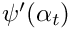is used for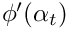and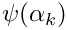until some value satisfies the test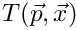and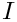then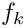is used from then on.
Interpolation Minimizer equations from Optimization Theory and Methods: Nonlinear Programming By Wenyu Sun, Ya-xiang Yuan (89-100).
Parameters
 [in] a_l first endpoint of interval,in Moore-Thuente (1994) [in] f_l value at first endpoint,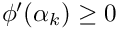in Moore-Thuente (1994) [in] g_l derivative at first endpoint,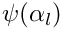in Moore-Thuente (1994) [in] a_u second endpoint of interval,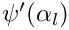in Moore-Thuente (1994) [in] f_u value at second endpoint,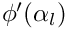in Moore-Thuente (1994) [in] g_u derivative at second endpoint,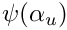in Moore-Thuente (1994) [in] a_t previous trial value,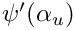in Moore-Thuente (1994) [in] f_t value at previous trial value,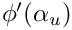in Moore-Thuente (1994) [in] g_t derivative at previous trial value,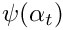in Moore-Thuente (1994)
Returns
new trial value

Definition at line 543 of file ndt.hpp.

## ◆ updateDerivatives()

template<typename PointSource , typename PointTarget , typename Scalar >
 double pcl::NormalDistributionsTransform< PointSource, PointTarget, Scalar >::updateDerivatives ( Eigen::Matrix< double, 6, 1 > & score_gradient, Eigen::Matrix< double, 6, 6 > & hessian, const Eigen::Vector3d & x_trans, const Eigen::Matrix3d & c_inv, bool compute_hessian = true ) const
protected

Compute individual point contributions to derivatives of likelihood function w.r.t.

the transformation vector.

Note
Equation 6.10, 6.12 and 6.13 [Magnusson 2009].
Parameters
 [in,out] score_gradient the gradient vector of the likelihood function w.r.t. the transformation vector [in,out] hessian the hessian matrix of the likelihood function w.r.t. the transformation vector [in] x_trans transformed point minus mean of occupied covariance voxel [in] c_inv covariance of occupied covariance voxel [in] compute_hessian flag to calculate hessian, unnecessary for step calculation.

Definition at line 373 of file ndt.hpp.

## ◆ updateHessian()

template<typename PointSource , typename PointTarget , typename Scalar >
 void pcl::NormalDistributionsTransform< PointSource, PointTarget, Scalar >::updateHessian ( Eigen::Matrix< double, 6, 6 > & hessian, const Eigen::Vector3d & x_trans, const Eigen::Matrix3d & c_inv ) const
protected

Compute individual point contributions to hessian of likelihood function w.r.t.

the transformation vector.

Note
Equation 6.13 [Magnusson 2009].
Parameters
 [in,out] hessian the hessian matrix of the likelihood function w.r.t. the transformation vector [in] x_trans transformed point minus mean of occupied covariance voxel [in] c_inv covariance of occupied covariance voxel

Definition at line 463 of file ndt.hpp.

## ◆ updateIntervalMT()

template<typename PointSource , typename PointTarget , typename Scalar >
 bool pcl::NormalDistributionsTransform< PointSource, PointTarget, Scalar >::updateIntervalMT ( double & a_l, double & f_l, double & g_l, double & a_u, double & f_u, double & g_u, double a_t, double f_t, double g_t ) const
protected

Update interval of possible step lengths for More-Thuente method,in More-Thuente (1994)

Note
Updating Algorithm until some value satisfiesandand Modified Updating Algorithm from then on [More, Thuente 1994].
Parameters
 [in,out] a_l first endpoint of interval,in Moore-Thuente (1994) [in,out] f_l value at first endpoint,in Moore-Thuente (1994),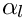for Update Algorithm and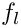for Modified Update Algorithm [in,out] g_l derivative at first endpoint,in Moore-Thuente (1994),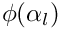for Update Algorithm and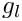for Modified Update Algorithm [in,out] a_u second endpoint of interval,in Moore-Thuente (1994) [in,out] f_u value at second endpoint,in Moore-Thuente (1994),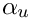for Update Algorithm and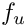for Modified Update Algorithm [in,out] g_u derivative at second endpoint,in Moore-Thuente (1994),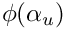for Update Algorithm and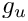for Modified Update Algorithm [in] a_t trial value,in Moore-Thuente (1994) [in] f_t value at trial value,in Moore-Thuente (1994),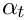for Update Algorithm and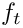for Modified Update Algorithm [in] g_t derivative at trial value,in Moore-Thuente (1994),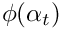for Update Algorithm and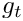for Modified Update Algorithm
Returns
if interval converges

Definition at line 498 of file ndt.hpp.

## ◆

 union { ... }

The likelihood score of the transform applied to the input cloud, Equation 6.9 and 6.10 [Magnusson 2009].

## ◆ angular_hessian_

template<typename PointSource , typename PointTarget , typename Scalar = float>
 Eigen::Matrix pcl::NormalDistributionsTransform< PointSource, PointTarget, Scalar >::angular_hessian_
protected

Precomputed Angular Hessian.

The precomputed angular derivatives for the hessian of a transformation vector, Equation 6.19 [Magnusson 2009].

Definition at line 594 of file ndt.h.

## ◆ angular_jacobian_

template<typename PointSource , typename PointTarget , typename Scalar = float>
 Eigen::Matrix pcl::NormalDistributionsTransform< PointSource, PointTarget, Scalar >::angular_jacobian_
protected

The precomputed angular derivatives for the jacobian of a transformation vector, Equation 6.19 [Magnusson 2009].

Definition at line 587 of file ndt.h.

## ◆ gauss_d1_

template<typename PointSource , typename PointTarget , typename Scalar = float>
 double pcl::NormalDistributionsTransform< PointSource, PointTarget, Scalar >::gauss_d1_
protected

The normalization constants used fit the point distribution to a normal distribution, Equation 6.8 [Magnusson 2009].

Definition at line 570 of file ndt.h.

## ◆ gauss_d2_

template<typename PointSource , typename PointTarget , typename Scalar = float>
 double pcl::NormalDistributionsTransform< PointSource, PointTarget, Scalar >::gauss_d2_
protected

Definition at line 570 of file ndt.h.

## ◆ outlier_ratio_

template<typename PointSource , typename PointTarget , typename Scalar = float>
 double pcl::NormalDistributionsTransform< PointSource, PointTarget, Scalar >::outlier_ratio_
protected

The ratio of outliers of points w.r.t.

a normal distribution, Equation 6.7 [Magnusson 2009].

Definition at line 566 of file ndt.h.

## ◆ point_hessian_

template<typename PointSource , typename PointTarget , typename Scalar = float>
 Eigen::Matrix pcl::NormalDistributionsTransform< PointSource, PointTarget, Scalar >::point_hessian_
protected

The second order derivative of the transformation of a point w.r.t.

the transform vector,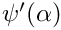in Equation 6.20 [Magnusson 2009].

Definition at line 604 of file ndt.h.

## ◆ point_jacobian_

template<typename PointSource , typename PointTarget , typename Scalar = float>
 Eigen::Matrix pcl::NormalDistributionsTransform< PointSource, PointTarget, Scalar >::point_jacobian_
protected

The first order derivative of the transformation of a point w.r.t.

the transform vector,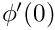in Equation 6.18 [Magnusson 2009].

Definition at line 599 of file ndt.h.

## ◆ resolution_

template<typename PointSource , typename PointTarget , typename Scalar = float>
 float pcl::NormalDistributionsTransform< PointSource, PointTarget, Scalar >::resolution_
protected

## ◆ step_size_

template<typename PointSource , typename PointTarget , typename Scalar = float>
 double pcl::NormalDistributionsTransform< PointSource, PointTarget, Scalar >::step_size_
protected

The maximum step length.

Definition at line 562 of file ndt.h.

## ◆ target_cells_

template<typename PointSource , typename PointTarget , typename Scalar = float>
 TargetGrid pcl::NormalDistributionsTransform< PointSource, PointTarget, Scalar >::target_cells_
protected

The voxel grid generated from target cloud containing point means and covariances.

Definition at line 556 of file ndt.h.

## ◆ trans_likelihood_

template<typename PointSource , typename PointTarget , typename Scalar = float>
 double pcl::NormalDistributionsTransform< PointSource, PointTarget, Scalar >::trans_likelihood_

## ◆ trans_probability_

template<typename PointSource , typename PointTarget , typename Scalar = float>
 double pcl::NormalDistributionsTransform< PointSource, PointTarget, Scalar >::trans_probability_
Deprecated:
Scheduled for removal in version 1 . 16 : "trans_probability_ has been renamed to trans_likelihood_."

Definition at line 578 of file ndt.h.

The documentation for this class was generated from the following files: